This topic describes how to build models to predict the hazy weather based on the analysis of weather data that is collected in Beijing for one year. The models can be used to find out the pollutant that is most prone to cause hazy weather, which is measured based on the concentration of PM 2.5.

## Dataset

In the following sample experiment, the air quality data that is collected every hour in Beijing during 2016 is used. The following table describes the fields of the air quality data.
Field Data type Description
time STRING The date. This field is accurate to the day.
hour STRING The hour in which the data is collected.
pm2 STRING The PM 2.5 index.
pm10 STRING The PM 10 index.
so2 STRING The sulfur dioxide index.
co STRING The carbon monoxide index.
no2 STRING The nitrogen dioxide index.

## Procedure

1. Go to the Machine Learning Studio console.
1. Log on to the PAI console.
2. In the left-side navigation pane, choose Model Training > Studio-Modeling Visualization.
3. On the PAI Visualization Modeling page, find the project in which you want to create an experiment and click Machine Learning in the Operation column.2. Create an experiment.
1. In the left-side navigation pane, click Home.
2. In the Templates section, click Create below Air Quality Prediction.
3. In the New Experiment dialog box, set the experiment parameters. You can use default values for the parameters.
Parameter Description
Name The name of the experiment. Default value: Air Quality Prediction.
Project The project in which you want to create the experiment. You cannot change the value of this parameter.
Description The description of the experiment. Default value: Use machine learning algorithms to analyze the effects of nitrogen dioxide on hazy weather.
Save To The directory for storing the experiment. Default value: My Experiments.
4. Click OK.
5. Optional:Wait about 10 seconds. Then, click Experiments in the left-side navigation pane.
6. Optional:Click Air Quality Prediction_XX under My Experiments. The canvas of the experiment appears.
My Experiments is the directory for storing the experiment that you created and Air Quality Prediction_XX is the name of the experiment. In the experiment name, _XX is the ID that the system automatically creates for the experiment.
7. View the components of the experiment on the canvas, as shown in the following figure. The system automatically creates the experiment based on the preset template.Area No. Description
1 The components in this area read and preprocess data.
1. The pai_online_project.wumai_data-1 component reads the source data.
2. The Data Type Conversion-1 component converts the source data in the STRING type to the DOUBLE type.
3. The SQL Script-1 component converts the values in the label column to binary values of 0 or 1. In this experiment, the pm2 column is the label column. In the pm2 column, values greater than 200 indicate heavy hazy weather. The SQL Script-1 component marks the values greater than 200 in the pm2 column as 1 and the values that are equal to or smaller than 200 as 0 by executing the following SQL statement:
``select time,hour,(case when pm2>200 then 1 else 0 end),pm10,so2,co,no2 from \${t1};``
4. The Normalization-1 component converts pollutant concentrations with different units to values without units.
2 The components in this area perform statistical analysis:
1. The Histogram (Multiple Columns)-1 component visualizes the distribution of each pollutant.
For example, the following figure shows that the interval where most of the PM 2.5 concentrations fall is 11.74 to 15.61. The total number of PM 2.5 concentrations in this interval is 430.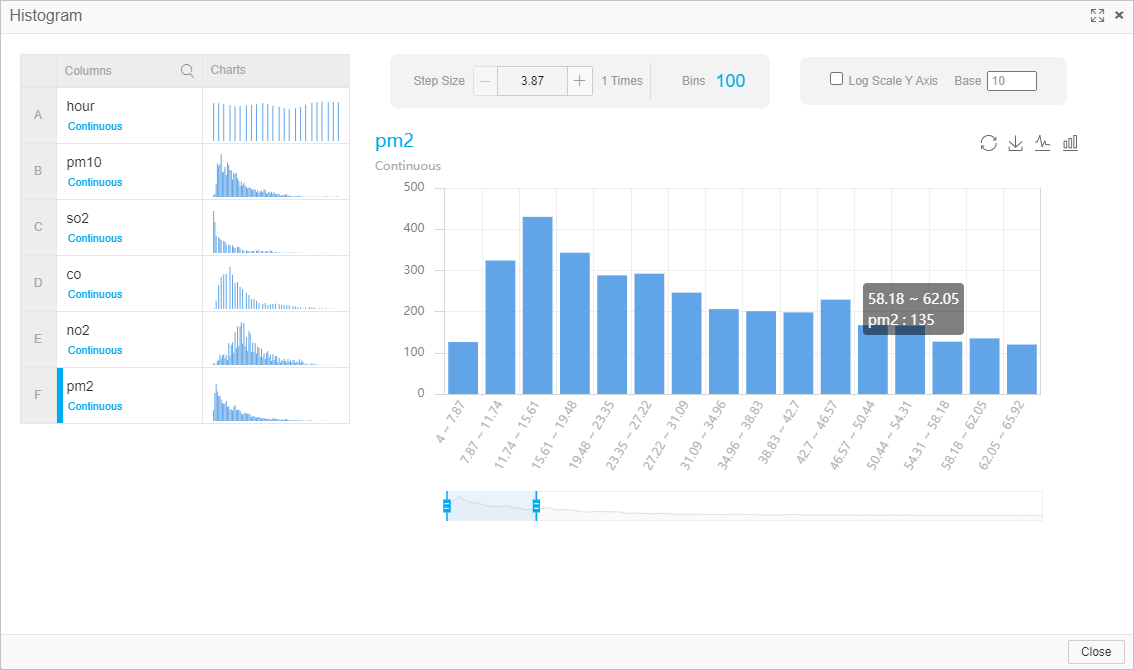2. The Data Pivoting-1 component visualizes how the concentration of each pollutant affects the prediction result.
For example, the following figure shows the data of the nitrogen dioxide concentration. When the nitrogen dioxide concentration falls in the interval of 112.33 to 113.9, seven values of the label column are converted to 0 and nine are converted to 1. This indicates that when the nitrogen dioxide concentration falls in the interval of 112.33 to 113.9, the occurrence probability of heavy hazy weather is high. The entropy and Gini index are the criteria based on which the information gain is calculated. The greater the entropy and Gini index values of an interval are, the greater the impact of the concentrations in the interval on the air quality is.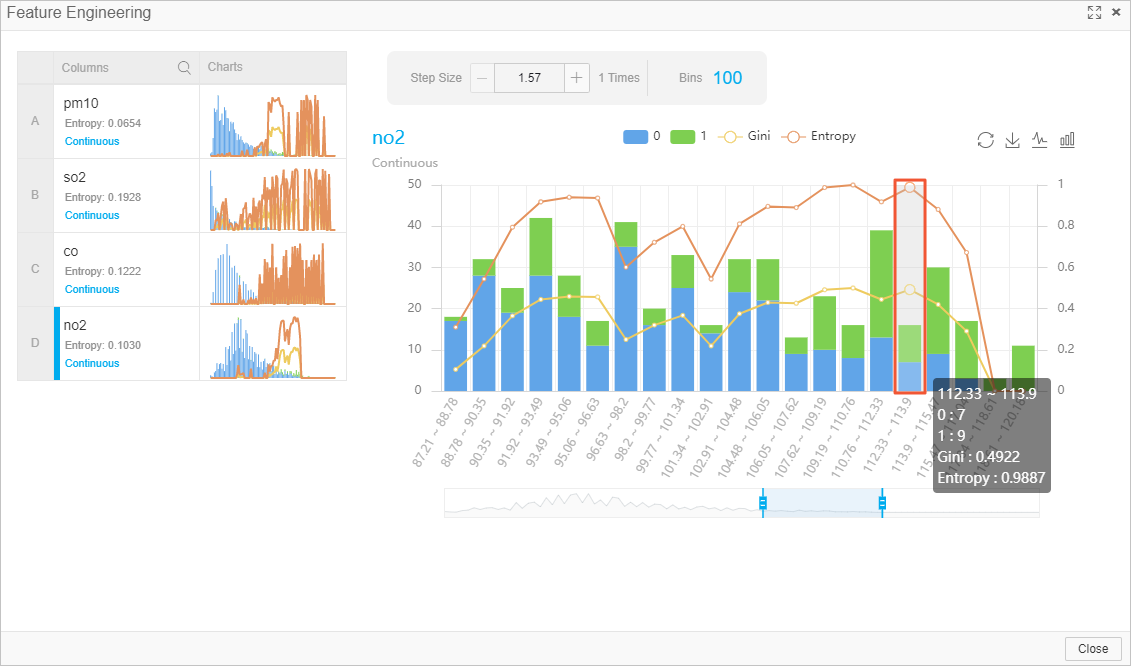3 The components in this area train models and make predictions. In this experiment, the Random Forest-1 and Logistic Regression for Binary Classification-1 components train the models.
4 The components in this area evaluate the models.
3. Run the experiment and view the result.
1. In the top toolbar of the canvas, click Run.
2. After the experiment is run, right-click the Binary Classification Evaluation-1 component on the canvas and select View Evaluation Report.
3. In the Evaluation Report dialog box, click the Charts tab to view the prediction results of the models that are trained by the Random Forest-1 component.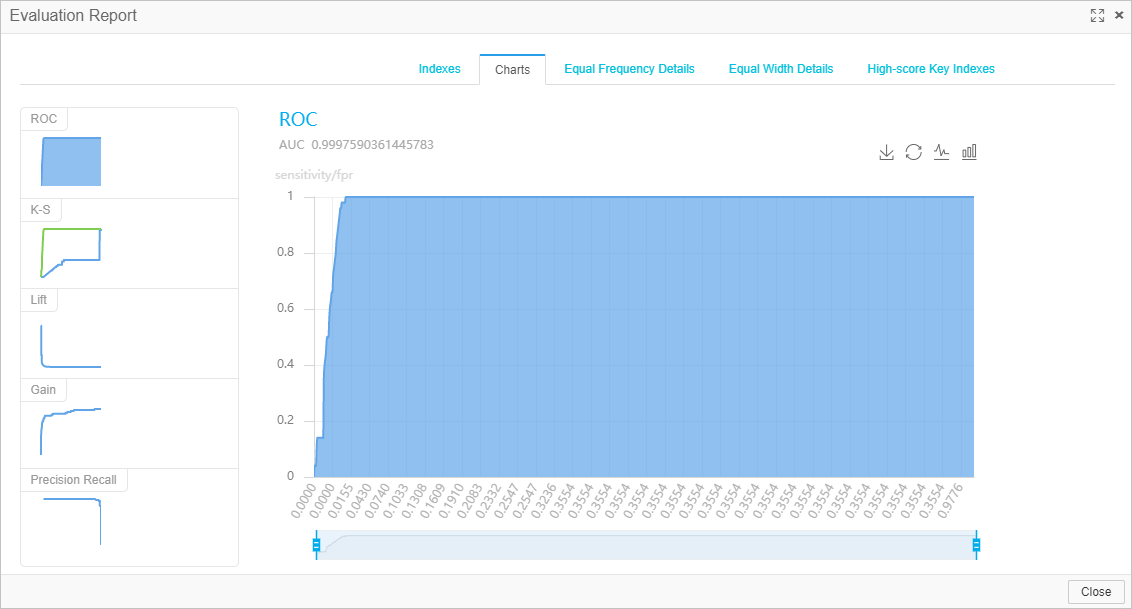The area under curve (AUC) value in the preceding figure indicates that the accuracy of the trained model for air quality prediction is higher than 99%. This model is trained by the Random Forest-1 component. You can right-click the Random Forest-1 component on the canvas and choose Model Option > Show Model to view the prediction model.
4. Right-click the Binary Classification Evaluation-2 component on the canvas and select View Evaluation Report.
5. In the Evaluation Report dialog box, click the Charts tab to view the prediction result of the model that is trained by the Logistic Regression for Binary Classification-1 component.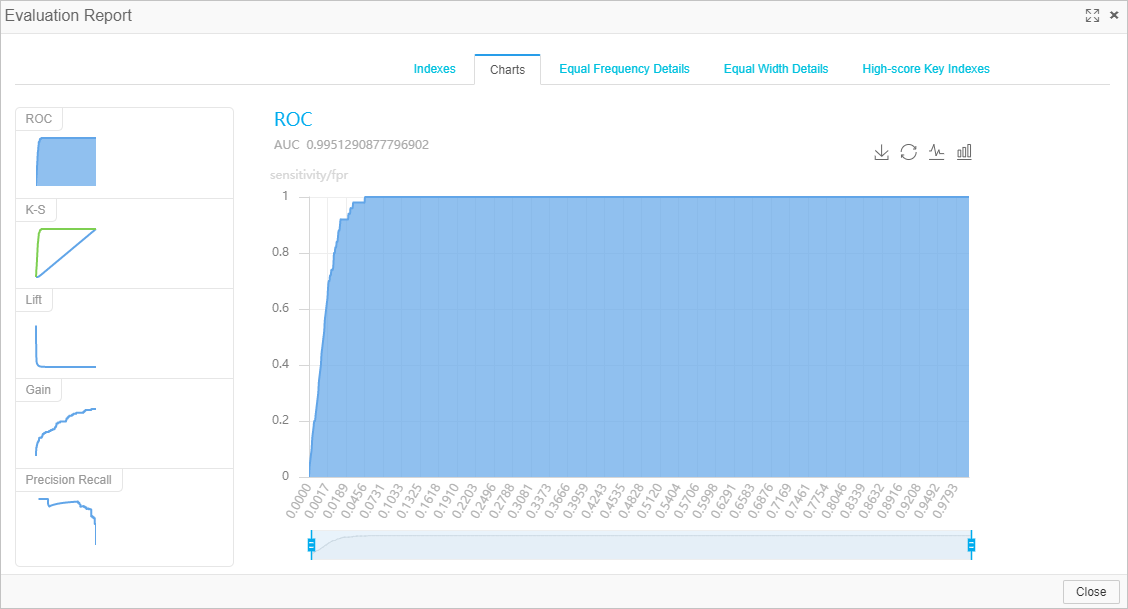The AUC value in the preceding figure indicates the accuracy of the model for the hazy weather prediction is higher than 99%. This model is trained by the Logistic Regression for Binary Classification-1 component. You can right-click the Logistic Regression for Binary Classification-1 component on the canvas and choose Model Option > Show Model to view the prediction model. The following figure shows the prediction model.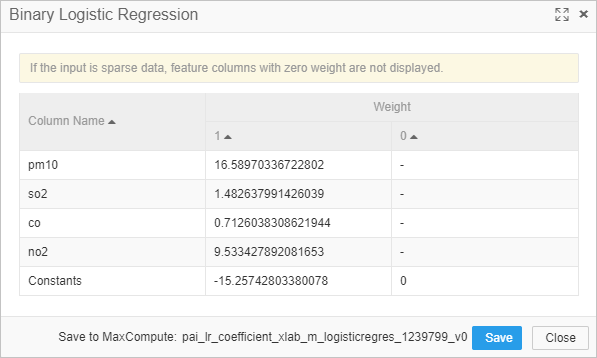The higher the weight of a normalized pollutant index is, the greater the impact of the index on the prediction result is. A positive normalized value of a pollutant index indicates a positive correlation between the pollutant and the hazy weather. A negative normalized value of a pollutant index indicates a negative correlation between the pollutant and the hazy weather. As shown in the preceding figure, the pollutants that have the greatest weights are PM 10 and nitrogen dioxides that are positively correlated with the air quality. PM 10 and PM 2.5 are similar excluding the particle size. The impact of PM 10 can be ignored. Therefore, nitrogen dioxide is most prone to cause hazy weather.